Ex 5.2

Chapter 5 Class 11 Complex Numbers
Serial order wise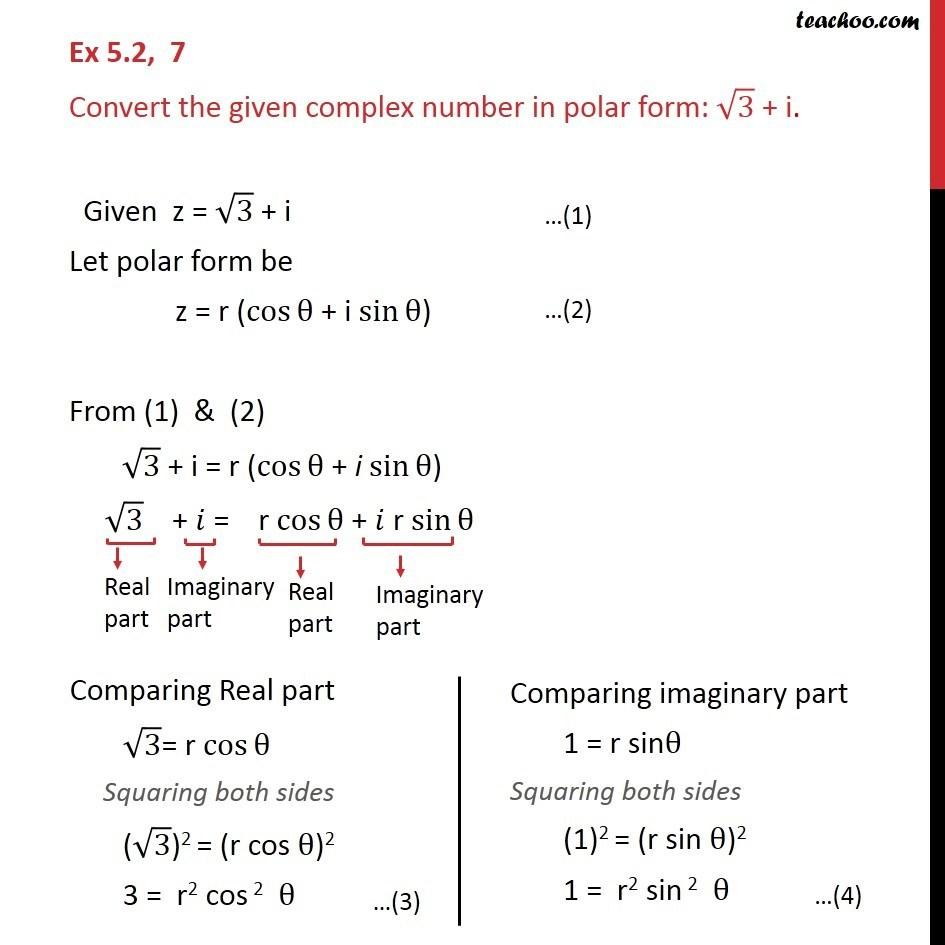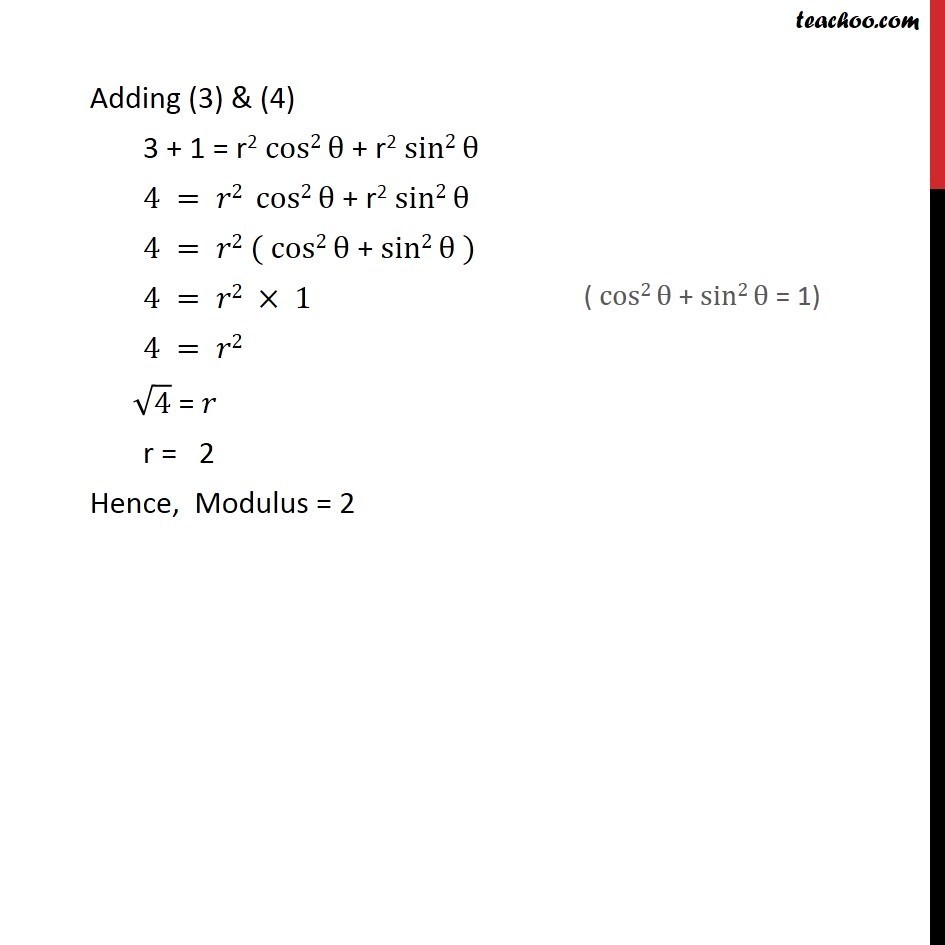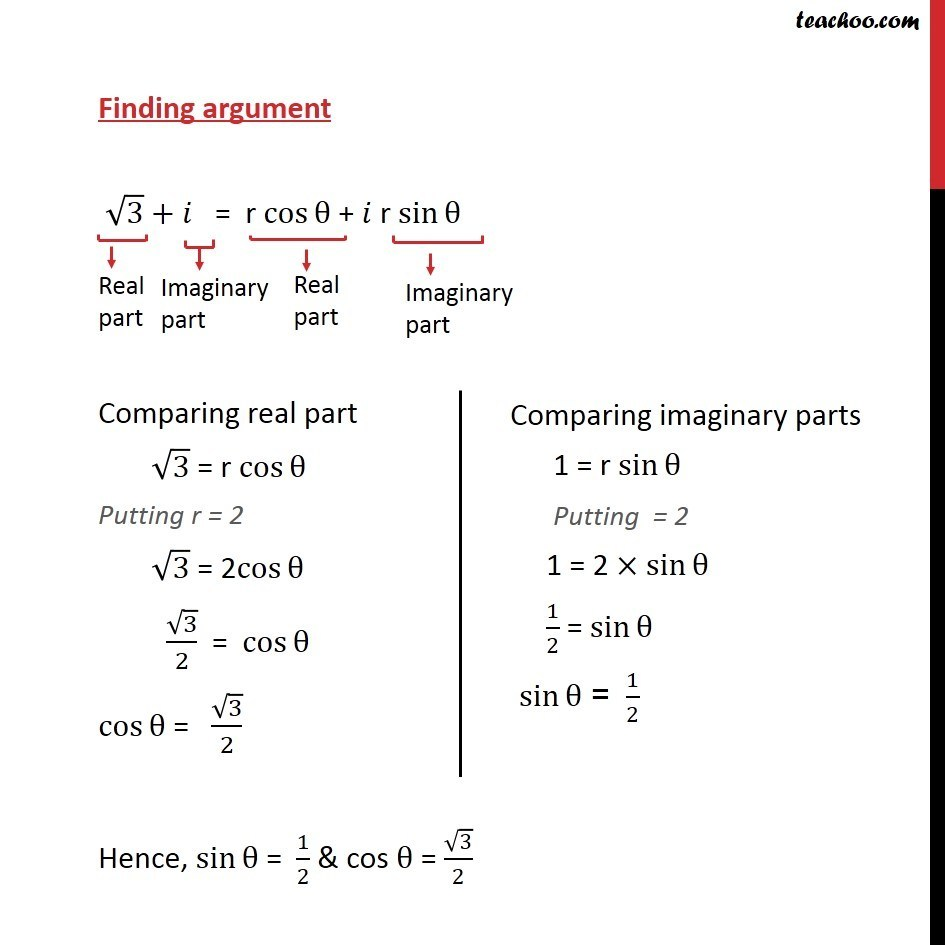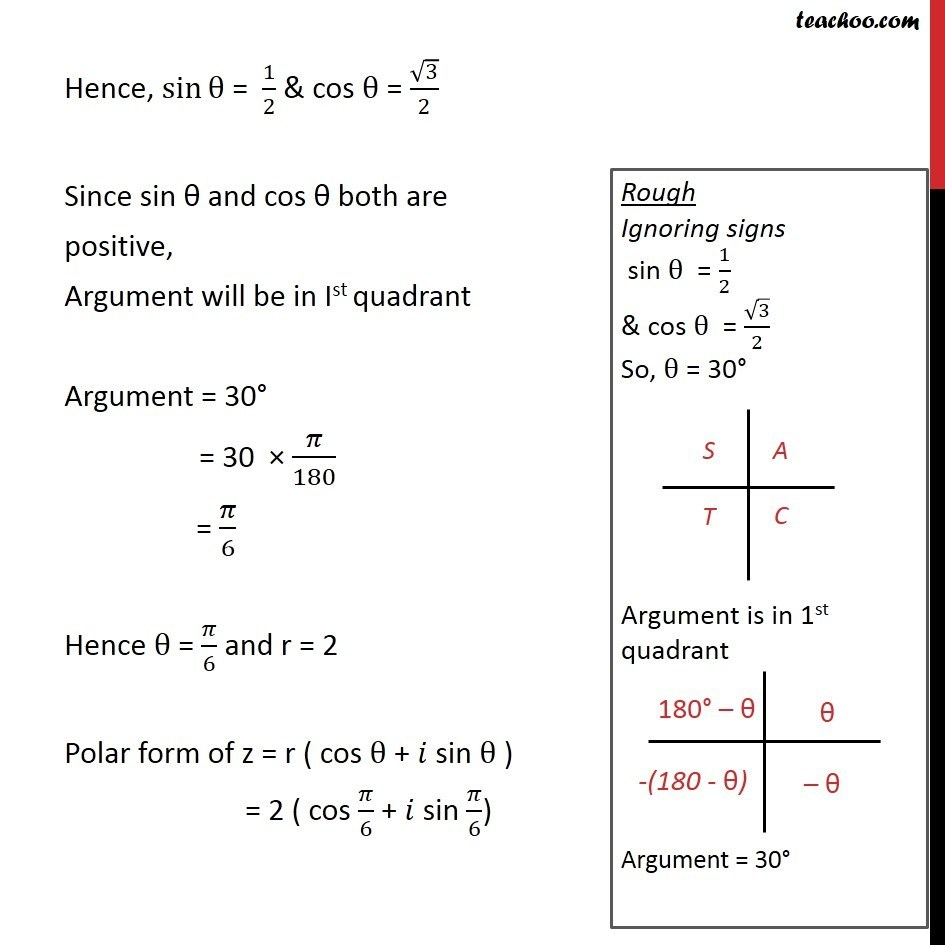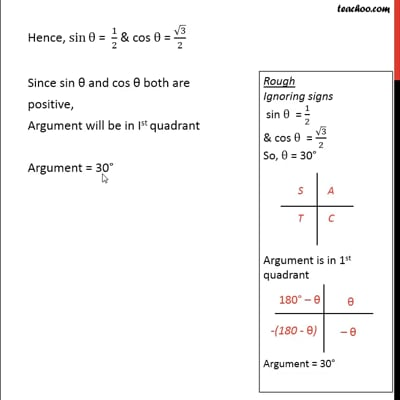This video is only available for Teachoo black users

Introducing your new favourite teacher - Teachoo Black, at only ₹83 per month

### Transcript

Ex 5.2, 7 Convert the given complex number in polar form: 3 + i. Given z = 3 + i Let polar form be z = r (cos + i sin ) From (1) & (2) 3 + i = r (cos + i sin ) 3 + = r cos + r sin Adding (3) & (4) 3 + 1 = r2 cos2 + r2 sin2 4 = 2 cos2 + r2 sin2 4 = 2 ( cos2 + sin2 ) 4 = 2 1 4 = 2 4 = r = 2 Hence, Modulus = 2 Finding argument 3 + = r cos + r sin Comparing real part 3 = r cos Putting r = 2 3 = 2cos 3/2 = cos cos = 3/2 Hence, sin = 1/2 & cos = 3/2 Hence, sin = 1/2 & cos = 3/2 Since sin and cos both are positive, Argument will be in Ist quadrant Argument = 30 = 30 /180 = /6 Hence = /6 and r = 2 Polar form of z = r ( cos + sin ) = 2 ( cos /6 + sin /6)